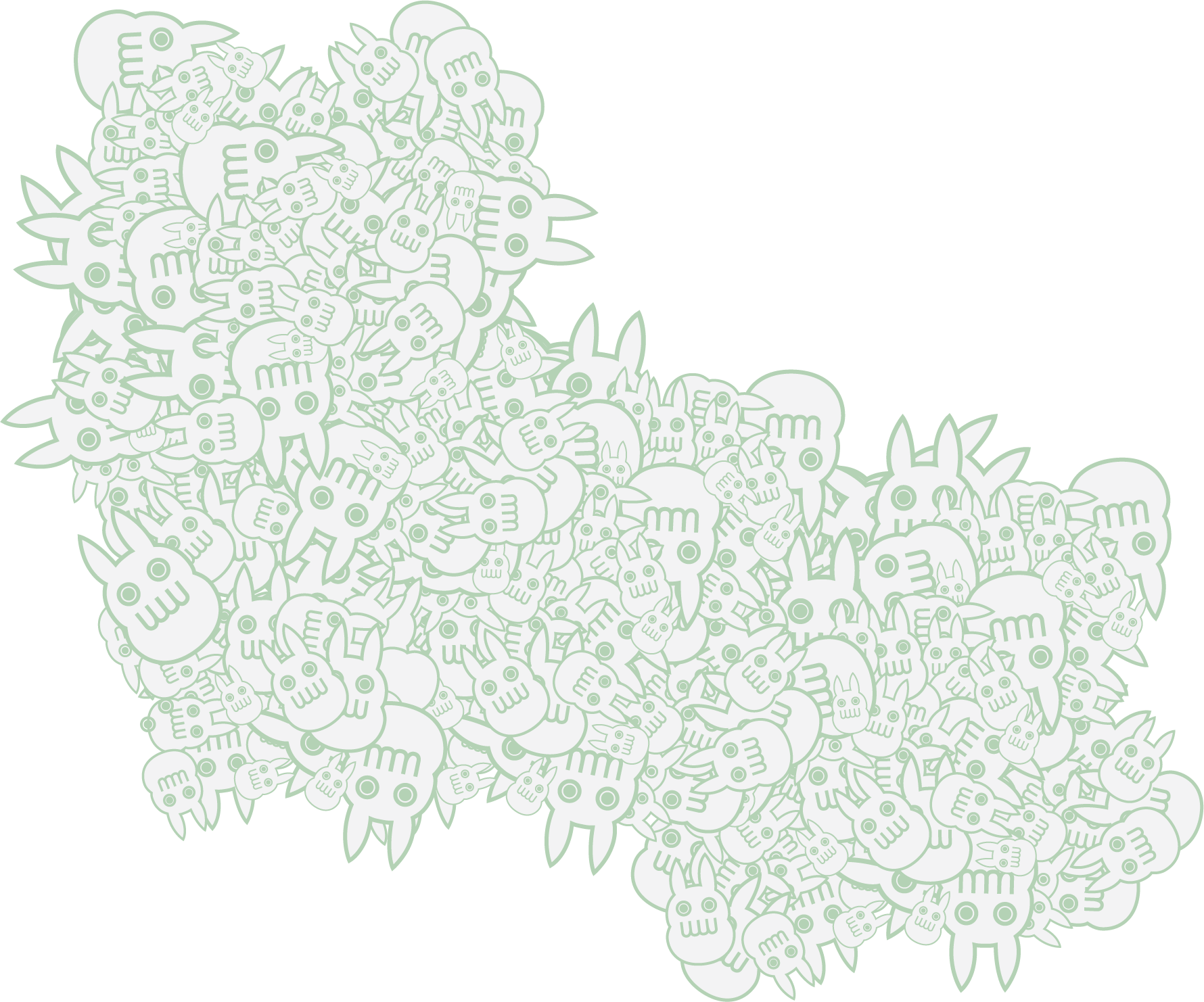Search

# ICM Week 1 - shapes and color with p5.js

Here's my first sketch drawn in p5.js:Loch Ness Monster

## What worked

I definitely wanted to use some form of gradient for this first drawing, initially wanting to contain the gradient within a shape, but after looking at some sample code I realized that might have been too ambitious for a first drawing. Having worked in Processing in the past, I wrongly assumed that I could muster up some of my previous knowledge and create what I had originally intended. Needless to say - I remember virtually nothing, but it was also over 8 years ago.

I was able to adapt some code from the p5.js examples page for creating linear gradients. After reading a bit about how the lerpColor() function works, I was able to adapt what code I needed to make my simple gradient work - with some trial and error of course.

## What didn't work

Here's the part where I talk about my brilliant idea to use the trig functions in p5 in order to make some thick lined waves to make up the water for Nessy to swim in. Well, after looking at some of the reference guides, I realized that I also didn't remember anything from trigonometry OR using trig functions in Processing. And of course all the examples were animated and super cool looking, making the code longer and more complicated.

So...after a few hours of tinkering and not wanting to abandon my idea, I turned to brute force. Which is when the madness started. As you'll see below, my code is very long and veeeeeeeeery slow. Near the end of the sketch it started taking about 5 seconds just to edit a single number value.

I also attempted some linear arrays in order to save myself some time and lines of code, knowing full well how SLOW things would get. But sadly I was having trouble getting arrays to work, it was also around midnight at the time and my brain had decided it was time to stop trying to interpret loops and "let/for" statements.

## What I learned

Mostly that I don't remember any of my previous experience working in a similar language (Processing), but I remember how frustrating and fun the process is - and how satisfying the results are. Yes, I could have made this in Adobe Illustrator probably 50x faster and better than using p5.js, but I MADE THIS IMAGE BY ONLY TYPING LINES OF TEXT. That will never NOT be satisfying and cool and rewarding.

## Code ----------------------------------------------------Long:

```// Gradient code taken from p5.js Linear Gradient
// The lerpColor() function is useful for interpolating between two colors

// Constants
let c1, c2;

function setup() {
createCanvas(600, 300);

// Define colors
c1 = color(252, 3, 190);
c2 = color(50, 102, 200);

}

function draw() {

}

function setGradient(x, y, w, h, c1, c2, axis) {

for (let i = y; i <= y + h; i++) {
let inter = map(i, y, y + h, 0, 1);
let c = lerpColor(c1, c2, inter);
stroke(c);
line(x, i, x + w, i);

//Sun
fill(0)
stroke(244, 3, 252, 2)
strokeWeight(15)
circle(300, -10, 450)
//scales
fill(252, 177, 3)
noStroke()
circle(70, 176, 20)
circle(100, 165, 20)
circle(135, 175, 20)
circle(158, 200, 20)
circle(172, 235, 20)
circle(222, 235, 20)
circle(245, 200, 20)
circle(285, 183, 20)
circle(330, 186, 20)
circle(364, 210, 20)
circle(378, 245, 20)
circle(500, 245, 20)
circle(485, 227, 20)

//monster
stroke(0, 207, 172);
strokeWeight(45);
noFill()
arc(100, 250, 100, 130, PI + QUARTER_PI, 0);
strokeWeight(40);
arc(300, 250, 120, 100, PI, 0);
strokeWeight(35);
arc(470, 260, 100, 100, PI + HALF_PI, TWO_PI);
//arc(470,160,100,100,HALF_PI,PI);

//darkgreen
stroke(119, 2, 173)
strokeWeight(26)
arc(99, 256, 82, 119, PI + QUARTER_PI, 0);
arc(300, 260, 100, 100, PI, 0);
strokeWeight(20)
arc(468, 255, 117, 106, PI + HALF_PI, TWO_PI);

//eye
strokeWeight(10)
stroke(0, 207, 172)
circle(52, 189, 5)
stroke(0, 0, 0)
strokeWeight(8)
point(53, 190)

strokeWeight(2)
arc(53, 190, 15, 15, 0, HALF_PI)

//mouth

stroke(0)
strokeWeight(3);
arc(68, 220, 30, 30, HALF_PI + QUARTER_PI, PI + QUARTER_PI)

//horn
stroke(0, 171, 125)
strokeWeight(7);
line(75, 140, 70, 172)
line(60, 150, 68, 160)

//nose
strokeWeight(10)
stroke(0, 207, 172)
circle(42, 210, 5)
stroke(0, 0, 0)
strokeWeight(2)
arc(46, 210, 15, 10, PI, PI + QUARTER_PI)

//water
noStroke()
fill(0, 177, 204);
rect(0, 260, 600, 30)
//water
noStroke()
fill(10, 20, 155);
rect(0, 280, 600, 30)

//wave1
stroke(25, 180, 255)
strokeWeight(8);
fill(0, 177, 204);
beginShape();
curveVertex(0, 260);
curveVertex(0, 260);
curveVertex(20, 240); //+20
curveVertex(70, 260); //+50
curveVertex(90, 240); //+20
curveVertex(140, 260); //+50
curveVertex(160, 240); //+20
curveVertex(210, 260);
curveVertex(230, 240); //+20
curveVertex(280, 260);
curveVertex(300, 240); //+20
curveVertex(350, 260);
curveVertex(370, 240); //+20
curveVertex(420, 260);
curveVertex(440, 240); //+20
curveVertex(490, 260);
curveVertex(510, 240); //+20
curveVertex(560, 260);
curveVertex(580, 240); //+20
curveVertex(630, 260);
curveVertex(630, 240); //+20
endShape();

//Wave2
stroke(10, 120, 255)
strokeWeight(8);
fill(10, 20, 155);
beginShape();
curveVertex(0, 280);
curveVertex(0, 280);
curveVertex(50, 260);
curveVertex(70, 280); //+20
curveVertex(120, 260); //+50
curveVertex(140, 280); //+20
curveVertex(190, 260); //+50
curveVertex(210, 280); //+20
curveVertex(260, 260); //+50
curveVertex(280, 280); //+20
curveVertex(330, 260); //+50
curveVertex(350, 280); //+20
curveVertex(400, 260); //+50
curveVertex(420, 280); //+20
curveVertex(470, 260); //+50
curveVertex(490, 280); //+20
curveVertex(540, 260); //+50
curveVertex(560, 280); //+20
curveVertex(610, 260); //+50
curveVertex(630, 280); //+20
curveVertex(630, 280); //+20
endShape();

//wave3
stroke(0, 75, 181)
strokeWeight(8);
fill(1, 51, 97);
beginShape();
curveVertex(0, 300);
curveVertex(5, 300);
curveVertex(20, 280); //+20
curveVertex(70, 300); //+50
curveVertex(90, 280); //+20
curveVertex(140, 300); //+50
curveVertex(160, 280); //+20
curveVertex(210, 300);
curveVertex(230, 280); //+20
curveVertex(280, 300);
curveVertex(300, 280); //+20
curveVertex(350, 300);
curveVertex(370, 280); //+20
curveVertex(420, 300);
curveVertex(440, 280); //+20
curveVertex(490, 300);
curveVertex(510, 280); //+20
curveVertex(560, 300);
curveVertex(580, 280); //+20
curveVertex(630, 300);
curveVertex(630, 300); //+20
endShape();
}
}
}```

end of line.

See All